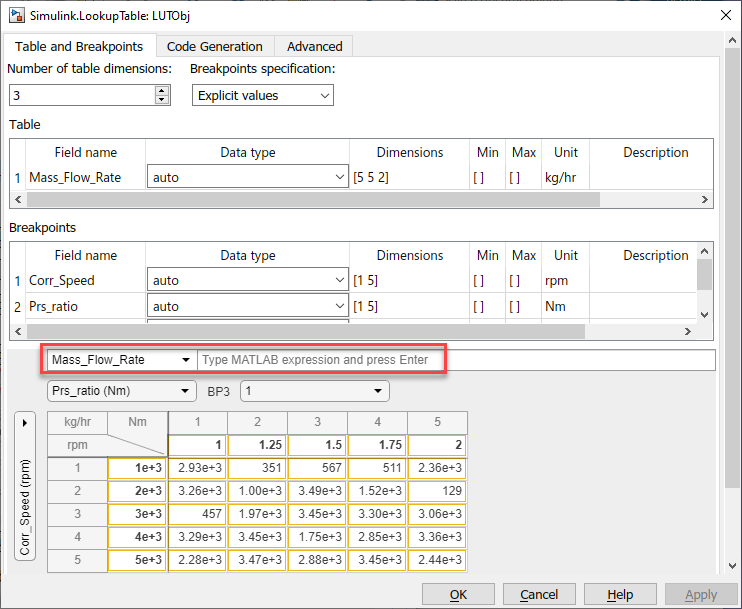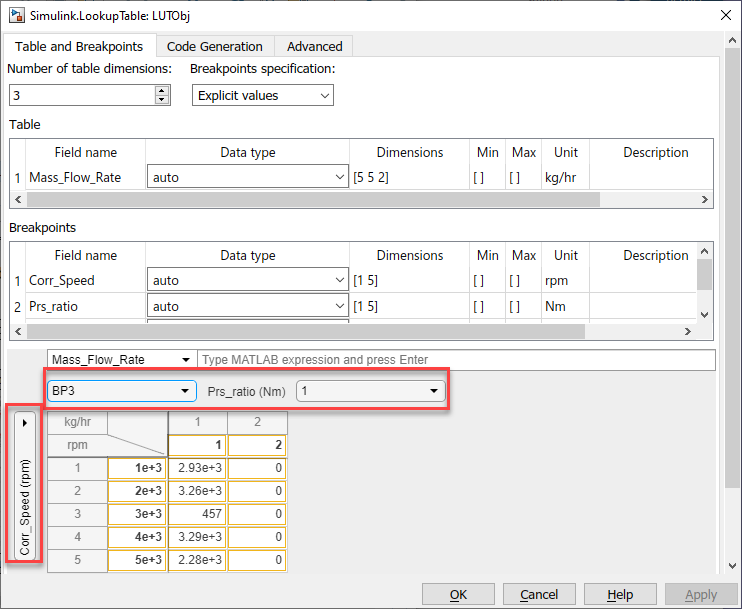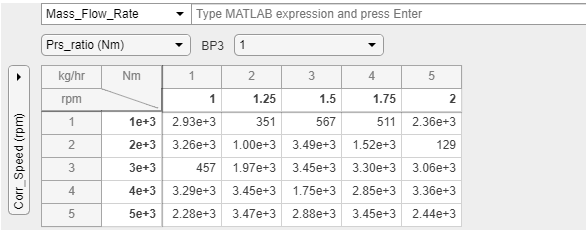## Edit Lookup Table Data with Lookup Table Spreadsheet

You can use a lookup table spreadsheet to view lookup table and breakpoint data. The lookup table spreadsheet is shared by multiple interfaces, including the `Simulink.LookupTable` property dialog box and the Lookup Table Editor. This topic and its examples are presented using the `Simulink.LookupTable` property dialog box. Here is lookup table data spreadsheet as seen through the `Simulink.LookupTable` property dialog box. The spreadsheet is highlighted.When the Breakpoints specification property is set to `Explicit values`, use the lookup table spreadsheet at the bottom to:

• View, add, manipulate, and remove table and breakpoint data.

• Create or modify table or breakpoint data using MATLAB® expressions.

Alternatively, you can copy and paste the contents of a Microsoft® Excel® spreadsheet or any spreadsheet application.• View and edit 2-D slices of data from multiple dimensions.This topic describes:

• Supported data types for the lookup table spreadsheet

• How to create, edit, and view table and breakpoint data in the tabular interface

• How to view multidimensional 2-D slices

• How the tabular interface handles data overflows

• How the tabular interface handles invalid data

• Supported keyboard shortcuts

### Lookup Table Spreadsheet Data Type Support

The spreadsheet supports these data types:

• Built-in data types (`int8`, `uint8`, `int16`, `uint16`, `int32`, `uint32`, `double`, `single`, `boolean`)

• Fixed-point data types

• `uint64`, `int64`

• `half`

It does not support other data types that Simulink® supports.

### Create `Simulink.LookupTable` Objects

To start working with a `Simulink.LookupTable` object, create one at the MATLAB command line:

`LUTObj = Simulink.LookupTable;`

### How to Open the `Simulink.LookupTable` Object Property Dialog Box

To work with the `Simulink.LookupTable` object, start its property dialog box in one of these ways from the MATLAB Command Window:

• In the workspace, double-click the `Simulink.LookupTable` object.

• In the Model Explorer, double-click the object in the specific workspace for the object.

• In the command line, use the `open` function, for example:

`open LUTObj`

### Create Table and Breakpoint Data

Generate data and manipulate it using standard table editing actions. You can generate lookup table data in multiple ways, such as in the workspace, from Microsoft Excel, and so forth, and copy that data into the lookup table spreadsheet. For `Simulink.LookupTable` objects, you can also generate data from within the property dialog box using MATLAB expressions.

This example describes how to create data in the MATLAB Command Window workspace and how to set up the property dialog box lookup table spreadsheet using an example with that data.

1. To create table and breakpoint data, at the MATLAB command line, type:

```LUTObj.Breakpoints(1).Value = fi(linspace(1000,5000,5),1,16,2); LUTObj.Breakpoints(2).Value = single(linspace(1,2,5)); LUTObj.Table.Value = uint16(rand(5,5)*60*60);```
2. Set up the Unit and Field name properties for the object.

```LUTObj.Breakpoints(1).Unit = 'rpm'; LUTObj.Breakpoints(2).Unit = 'Nm'; LUTObj.Table.Unit = 'kg/hr'; LUTObj.Breakpoints(1).FieldName = 'Corr_Speed'; LUTObj.Breakpoints(2).FieldName = 'Prs_ratio'; LUTObj.Table.FieldName = 'Mass_Flow_Rate';```
3. Open the property dialog box.

`open LUTObj;`4. From within the tabular area, you can perform typical table edits on particular breakpoint data, such as copying and pasting. Data that has been edited but not applied is outlined in yellow. When you are done, click Apply. Observe that the yellow outline for the data is gone.

Tip

To create this data directly in the lookup table spreadsheet:

1. Set Number of table dimensions to `2`.

2. Set the first breakpoint to `fi(linspace(1000,5000,5),1,16,2);`.

3. Set the second breakpoint to `single(linspace(1,2,5));`.

4. Set Table to `uint16(rand(5,5)*60*60);`.

### View Multidimensional Slices of Data

The spreadsheet lets you view and edit 2-D slices of data. This example describes how to use the slice selector to navigate through the 2-D slices of 3-D data.

To view a multidimensional slice, use the dropdown lists under the MATLAB expression parameter. There is a dropdown list for each breakpoint. To view a two-dimensional slice, choose a combination of the breakpoint data from the vertical and horizontal dropdown lists.

1. Change the table dimensions to `3`.2. In the tabular area at the bottom, change the horizontal breakpoint slice to `BP3`. Observe the changed view of the slice of data.### Edit Table and Breakpoint Data with MATLAB Expressions

Edit table and breakpoint data using standard table editing actions, such as cutting or copying and pasting, or directly editing table cells. You can also edit table and breakpoint data with MATLAB expressions.

This example shows how to replace the table and breakpoint using a MATLAB expression.

1. In the spreadsheet, select BP3 from the horizontal dimension dropdown list.

2. In the MATLAB expression field, enter `linspace(1000,9999,5)`.

3. Observe the changed view of the slice of data.### Edit Table and Breakpoint Data

You can perform standard table edits in the spreadsheet. This example shows how to apply the same value to multiple cells.

Replace a column of data with the same value.

1. In the spreadsheet, select row 1, column 2 of the table data.

2. Select the entire column by dragging down the column.

3. Enter `324`.

4. Press Ctrl+Enter.

5. Observe that the entire column of data is now `324`.Add a row to the table.

1. In the spreadsheet, right-click the last row of the table and select Insert > Insert Row Below.Delete a column in the table.

1. In the spreadsheet, right-click the last column of the table and select Delete > Delete Column.Select and paste a region in the table.

1. In the spreadsheet, select the top-left cell of your selection and drag to the bottom-right of your selection.2. Copy the selected area with Ctrl+C.

3. Select another area of the table. Paste the selected data with Ctrl+V.### Enable Heatmap for Table Data

You can view heatmaps for your data. Right-click any cell in the table and select Heatmap On.### Overflow Handling

The `Simulink.LookupTable` object property dialog box handles data overflows in the lookup table spreadsheet by automatically changing values to ones that do not cause overflows. For example, if you enter a value of `70000` in a cell for a data type of `uint16`, the property dialog box automatically changes the value to `65535`, which is the maximum number for `uint16`. For a list of supported `Simulink.LookupTable` object property dialog box data types, see Lookup Table Spreadsheet Data Type Support.

### Data Validation

The lookup table spreadsheet performs data validation when you enter a table cell value and press Enter. For example, if you enter `NaN`, `Inf` or `-Inf` as a table cell value, an error is displayed.

The MATLAB expression area also validates expressions. For example, if you enter an invalid expression, the text box is outlined in red and displays an error message on an expected expression. If you enter an expression for fixed-point data with a bias, the software evaluates and, as necessary, saturates the value to the nearest available fixed-point value before displaying the corrected value in the table.

### Lookup Table Spreadsheet Tabular Interface Shortcuts

ActionKey or Keys

Move to the table cell above current active cell.

Up Arrow

Move to table cell under current active cell.

Down Arrow

Move to the table cell to the right of the current active cell.

Right Arrow or Tab

Move to the table cell to the left side of current active cell.

Left Arrow or Tab+Shift

Move to the first table cell in a column.

Ctrl+Up Arrow

Move to the last table cell in a column.

Ctrl+Down Arrow

Move to the first table cell in a row.

Ctrl+Left Arrow

Move to the last table cell in a row.

Ctrl+Right Arrow

Selection

ActionKey or Keys

Select all.

Ctrl+A

Extend selection of the table cell above.

Shift+Up Arrow

Extend selection of the table cell underneath.

Shift+Down Arrow

Extend selection of the table cell to the right.

Shift+Right Arrow

Extend selection of the table cell to the left.

Shift+Left Arrow

Select all table cells in the row to the left, including the current cell.

Shift+Ctrl+Left Arrow

Select all table cells in the row to the right, including the current cell.

Shift+Ctrl+Right Arrow

Select all cells from the column to the top of the table, including the current cell.

Shift+Ctrl+Up Arrow

Select all cells from the column to the bottom of the table, including the current cell.

Shift+Ctrl+Down Arrow

Editor

ActionKey or Keys
Open or close table cell editor.Enter
Open table cell editor.F2
Cancel editing and close table cell editor.Esc
Clear table cell.Backspace or Delete
Copy table cell content.Ctrl+C
Cut table cell content.Ctrl+X
Paste table cell content.Ctrl+V
Fill all selected table cells with edited cell value.Ctrl+Enter
Undo.Ctrl+Z
Redo.Ctrl+Y

ActionKey or Keys

Move the selection to the next option in context menu.

Down Arrow

Move the selection to the previous option in context menu.

Up Arrow